# A model for the effect of ion pairing on an outer sphere electron transfer

Renat Nazmutdinov a, Paola Quaino b, Estefania Colombo b, Elizabeth Santos c and Wolfgang Schmickler*c
aKazan National Research Technological University, 420015 Kazan, Republic of Tatarstan, Russian Federation
bInstituto de Química Aplicada del Litoral, IQAL (UNL-CONICET), Santa Fe, Argentina
cInstitute of Theoretical Chemistry, Ulm University, Germany. E-mail: wolfgang.schmickler@uni-ulm.de

Received 8th April 2020 , Accepted 25th May 2020

First published on 26th May 2020

Ion pairing can strongly affect the rates of electron transfer reactions. To explain this effect, we propose a model Hamiltonian that describes the interactions between the pairing ion and the reactant, solvent and inner sphere reorganization, and bond breaking. Explicit expressions for the energies of the initial and final states, and for the energy of activation are derived in the weak adiabatic limit. The model is applied to the reduction of Cu(II) in the presence of chloride ions. For this purpose, the pertinent system parameters are obtained from density functional theory. Our model explains why the chloride ion enhances the rate of the first electron transfer in copper deposition.

## 1 Introduction

It is well known that ion pairing may effect both the rates and the thermodynamics of electron transfer reactions. In particular, this is true for the deposition of multivalent metal ions, where the first step is often a one-electron transfer. Indeed, the plating industry uses various recipes for the baths from which metal ions are deposited, and ion pairing certainly plays a part in these processes.1 For example, for copper deposition, baths containing both Cl ions and organic substances such as polyethylene glycol are commonly used.2

Considering the importance of ion pairing, there are surprisingly few theoretical investigations of its effect on electron transfer. There are a considerable number of molecular dynamics studies of ion pairing in the bulk of the solution, and we shall discuss a few of them below, but they describe only the initial or final state of an electron transfer. A notable exception is the elegant work of Nagy et al.,3 which combines experimental work on the catalysis of copper deposition by chloride ions with an investigation of the electronic overlap of the reactant with a copper electrode. They conclude that the presence of chloride ions enhances the overlap and thereby catalyzes electron transfer. The effect is mainly attributed to the fact that chloride complexes can approach the electrode to shorter distances than the pure aqua complexes.

In this work, we study the effects of ion pairing on electron transfer from a fundamental point of view. We start by proposing a model Hamiltonian for electron transfer in the presence of an ion that does not directly participate in the reaction. However, its interaction with the reactant changes during the electron transfer, and its mere presence affects the accompanying solvent and inner sphere reorganization. From the Hamiltonian, we derive an expression for the energy of activation for the reaction. We also consider the effect of bond breaking by including Saveant's model4,5 in an extended version of the Hamiltonian.

As an application of our model, we have considered the effect of chloride ions on copper deposition. For this purpose, we have performed DFT (density functional theory) calculations for several Cu(I) and Cu(II) complexes with and without chloride ions. While these calculations are somewhat approximate, they illustrate well how the model can be used, and they explain why the presence of chloride ions accelerates the deposition process.

## 2 The model Hamiltonian

We consider an outer sphere electron exchange between a redox center labelled a and a metal electrode in the presence of an ion, which does not participate directly in the reaction, but forms an ion pair with the reactant. Our model is an extension of the theory of adiabatic electron transfer reactions proposed by one of us,6 and has also similarities with a model for combined electron and proton transfer.7

The Hamiltonian consists of a number of terms, which we shall present in turn. Since we consider the transfer of one electron in the outer sphere, we can use the spinless version of the Anderson–Newns model8,9 for the reactant and its interaction with a metal electrode: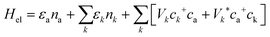(1)
The first two terms denote the reactant and the metal, and the last term effects electron exchange between the metal and the reactant with amplitude Vk. εk denotes the electronic energy of the metal states, nk is their number operator, and ck+ and ck are the creation and annihilation operators, respectively. The corresponding quantities for state a are εa, na, ca+ and ca. All energies εa and εk are measured with respect to the Fermi level of the metal.

Electron transfer is coupled to the reorganization of the solvent. Just like in Marcus10 and Hush11 theory, these modes are divided into a fast part, which is supposed to follow the electron transfer instantly, and a slow part. The former just renormalizes the electronic energy,12,13 and will not be discussed further. The latter is modeled as a phonon bath, whose modes we label by ν: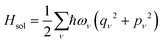(2)
where qν and pν are dimensionless coordinates and momenta, and ων is the frequency. These solvent modes interact with the charges of the reactant and of the ion that forms the pair. As usual, we take the interaction as linear and proportional to the charge. For simplicity, we assume that the charge number of the reactant is unity when the valence orbital is empty. So, we write the coupling terms as: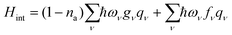(3)
Here, gν denotes the coupling constant of the reactant with the solvent modes, and fν denotes that of the ion. Since the latter does not change its charge state, we do not require its explicit value; it is contained in the coupling constant.

In addition, the interaction between the ion pair will change, and other inner sphere modes may be reorganized as well. We describe this by the term:

 Hpair = (1 − na)U1(x) + naU2(x) (4)
where x denotes a collection of variables. We assume that the two functions U1(x) and U2(x) have the same minimum value; any difference can be incorporated in εa. The sum of the terms in eqn (1)–(4) constitutes our model Hamiltonian.

In principle, electron transfer also involves a reorganization of the ionic atmosphere of the reactant. However, this effect is known to be weak and will not be discussed here.14

Our model makes no assumption about the strength of the interactions between the reactant and the metal, and can be applied to catalytic reactions as well. Here, however, we consider the outer sphere case. In the spirit of Marcus theory,10 we assume that the interaction between the reactant and the metal is sufficiently strong to assure adiabaticiy, but so small that it does not affect the energy. In addition, we assume that all the degrees of freedoms that are reorganized during the reaction are classical; other possibilities will be discussed below.

The energy of the system is a function of the coordinates qν and x. The stationary points on this surface are of particular importance; in the so-called normal region, where both the initial and the final points are stable, there are three such points corresponding to the initial, the transition, and the final states. Using Hellman–Feynman theorem, the conditions for stationary points are: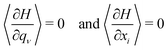(5)
In Marcus theory, the inner sphere modes are treated by the same harmonic approximation as the solvent modes. In this case, they can be included in the latter, i.e. the set qν then includes xi. In order to compare our results with those of Marcus theory, we shall follow this approximation at first, and return to the more general case later.

Thus, we consider only the first of eqn (5), which gives:

 qν = − (1 − 〈na〉)gν − fν (6)
At this stage, it is useful to define three energies of reorganization:7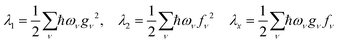(7)
where λ1 and λ2 characterize the coupling of the redox couple and of the ion to the solvent, respectively, and λx indicates the interference.

Considering electron transfer from the redox couple to the electrode, the initial state has 〈na〉 = 1, and the corresponding energy is:

 Ei = εa − λ2 (8)
Conversely, in the final state, we have 〈na〉 = 0, and the energy is:
 Ef = −λ1 − λ2 − 2λx (9)

The energy εa, taken with respect to the Fermi level, shifts with the applied electrode potential. At equilibrium:

 Ei = Ef andhence εa = −λ1 − 2λx (10)
In the absence of the complexing ion, εa = −λ1, so ion pairing has an effect on the equilibrium potential for the reaction. Of course, this does not come unexpectedly, but the advantage of our formalism is that it gives a quantitative expression.

Electron transfer happens at the saddle point; an easy way to determine the latter is to note that there, the electronic level of the reactant is at the Fermi level. For this purpose, we collect the terms in na and write: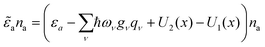(11)
where we have included the terms in U1(x) and U2(x) for further reference; they will be used in the next section. Settinga = 0 and using eqn (5) give: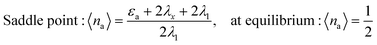(12)

Calculating the energy of activation is straightforward: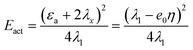(13)
where we have introduced the overpotential through εa = −λ1 − 2λx + e0η. This is the same expression as in normal Marcus theory without the complexing ion. Thus, the latter does not affect the expression for the energy of activation; of course it does affect the energy of reorganisation λ1 by its presence.

## 4 Bond breaking electron transfer

So far, we have not paid much attention to the term Hpair of eqn (4). If this denotes an inner sphere mode, it can be treated in the harmonic approximation, or if the exact curves are known, they can be incorporated into the formalism without difficulties. In particular, they will not affect the formal expression for the energy of activation.

A more interesting case arises when it refers to bond breaking or formation. Then, it is not a priori clear how this will be affected by the pair formation. To investigate this case, we adopt the model of Saveant4,5 and introduce the potentials:

 Hpair = (1 − na)D[e−2αx − 2e−αx] + naDe−2αx (14)
as proposed by Koper and Voth,15 and later adopted by our own group.16,17 The first term describes a Morse potential with binding energy D, and the second term contains only the repulsive part. These potentials contain the essentials of bond breaking, and permit analytical calculations. It is convenient to introduce: y = eαx. Then, the second part of eqn (5) for stationary points can be written in the compact form:
 y = (1 − 〈na〉) (15)
In the weak adiabatic limit, the energy of the occupied state with 〈na〉 = 1 remains unchanged:
 Ei = εa − λ2 (16)
while that for the empty state:
 Ef = −λ1 − λ2 − 2λx − D (17)
has been lowered by the binding energy. The calculation of the activation energy proceeds as before. Again, the formal result is not affected by the solvation of the ion: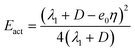(18)

In particular, at equilibrium, we have: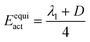(19)
Again, the ion pairing does not affect the formal expression of the activation energy, but those for the initial and final states. Of course, as stated before, the presence of the pairing ion affects the energy of reorganization by an excluded volume effect.

## 5 Effect of Cl− ions on the first step in copper deposition

The deposition of copper is known to be strongly affected by the presence of Cl ions, so we shall apply our formalism to this example, focusing on the transfer of the first electron in aqueous solutions. Chloride ions can replace water molecules as ligands in the nearest coordination sheath of d-metals. Therefore, we shall compare two different cases: electron transfer without Cl: Cu2+/Cu+, and with two Cl: [CuCl2]/[CuCl2]. For the complex with one chloride ion, CuCl+/CuCl, the reduced form has a very low solubility and forms an isolating deposit on the electrode surface. Therefore, it is generally avoided in praxis, and we shall not discuss it further.

In order to estimate both the changes in the thermodynamics and in the energy of activation caused by the presence of the chloride ions, we have performed DFT calculations for various complexes of copper with water and with Cl. Solvation effects were treated in the framework of a mixed molecular-continuum model; the technical details of the calculations are given in Appendix. These calculations were performed statically, without thermal average. However, because of the firm binding of water in the first and second solvation shells, this is not a severe limitation. In any case, we want to show how the formalism can be applied, and to understand trends rather than obtain absolute values.

Quantum chemical calculations for aqueous copper complexes have been performed by several other groups before.18–22 Our calculations complement these previous works by focusing on the energetics of the electron transfer.

### 5.1 Cu2+·aq/Cu+·aq

To investigate this reaction, we have considered copper complexes with 18 explicit water molecules. The rest of the solvent was described by an implicit solvation model (see Appendix). For the Cu2+ complex, we found two locally stable configurations with slightly different Jahn–Teller distortion.23 The most stable sixfold coordinated Cu2+ complex is shown at the left of Fig. 1; it has an axial Jahn–Teller distortion: r(Cu–Oeq) = 1.99 − 2.14 Å; r(Cu–Oax) = 2.29 Å. The subscripts eq and ax stand for equatorial and axial, respectively.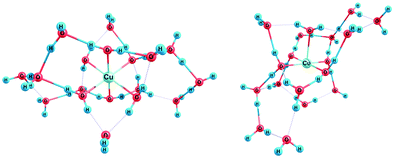Fig. 1 Optimized geometry of [Cu(H2O)18]2+ (oxidized state). Left: Sixfold, and right: fivefold coordinated complex.

Experimentally, a fivefold coordinated Cu2+ has also been observed by neutron diffraction24 and X-ray absorption spectroscopy.23,25–27 Further evidence in favor of this structure also follows from Car–Parrinello MD28 and ab initio molecular dynamics29,31 simulations. Therefore, we have also performed calculations for a fivefold coordinated complex (pyramidal structure) – see Fig. 2 right; according to our calculations, within DFT error, the Gibbs energies for both types of complexes are about the same (formally, the five-coordinated structure was found to be 0.072 eV more favorable). In the following, we consider only the fivefold complex. The calculated Cu–O bond lengths are in good agreement with experimental data;25 the difference amounts to 0.05 Å.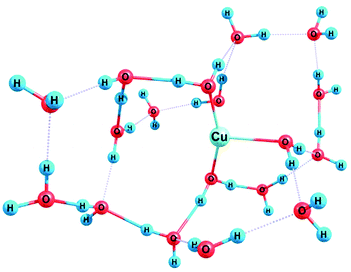Fig. 2 Optimized geometry of [Cu(H2O)18]+ (reduced state).

In qualitative accord with the literature,30 we have found that the reduced form [Cu(H2O)18]+ is only threefold coordinated. Two water molecules reveal the shortest Cu–O bond lengths, ca. 2 Å, while the bond of the Cu atom with the third molecule is elongated (2.32 Å). Blumberger et al. have reported a larger value (2.6–2.8 Å) for this bond length;29 this difference might be attributed to the shortcomings of our model. Ab initio calculations by Lee et al.17 point to a rather small difference of the hydration Gibbs energy between two-and threefold coordinated Cu+. Thus, the reduction can be considered to be accompanied by the breaking of at least two bonds. The calculated free energy of the reduction can be converted into the equilibrium potential by referring these values to the standard hydrogen electrode on the absolute scale. Unfortunately, the value of the latter is somewhat uncertain, 4.5 ± 0.2 V is a good estimate. Using the medium value, we obtain for the standard equilibrium potential a value of +0.22 V (fivefold Cu2+). The actual experimental value is 0.16 V.32 Considering the uncertainties of DFT and the approximate nature of the calculations, the agreement is acceptable. Actually, we are less interested in obtaining the absolute value but in the changes caused by complexation with the chloride ion. The energetics of the reorganization will be discussed below.

### 5.2 CuCl2·aq/CuCl2−·aq

For the CuCl2 complex in aqueous solutions, we found four different geometries with energy differences of less than 0.2 eV. The optimum geometry is shown on the left of Fig. 3; it has the form of a [CuCl2-trans](fivefold) complex with the shape of a pyramid. The Gibbs energy of the process Cu2+(aq) + 2 Cl(aq) = CuCl2(aq) is −0.55 eV, so that the addition of two chloride ions is favorable.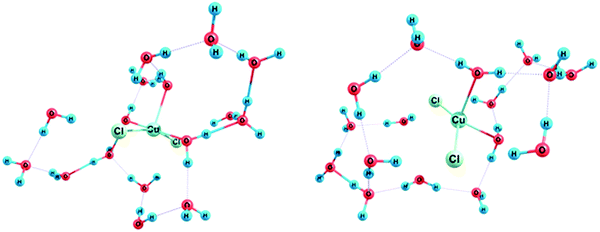Fig. 3 Left: Optimized geometry of [CuCl2(H2O)16] (oxidized state), and right: reduced state.

For the reduced form of this complex, we found two different forms: (i) and (ii). The form (i) looks like an ionic associate, [Cu(H2O)2]+2Cl, where the ions are not separated by water molecules. In contrast, the form (ii) is a tetrahedral four-coordinated complex [CuCl2(H2O)2] with the bond lengths r(Cu–Cl) = 2.33–2.34 Å and r(Cu–O) = 2.28–2.33 Å. The form (ii) is more stable by a Gibbs energy of 0.22 eV. Thus, the reduction entails the breaking of one bond, As shown by our calculations, the reduced form (Fig. 3, right) has no negative frequencies and can be considered as a local minimum of the multidimensional potential energy surface. We treat this form as a final state in the elementary act of electron transfer. This does not exclude, however, further transformation of the complex in subsequent chemical steps. The equilibrium potential was estimated to be +0.07 V, i.e., within DFT error, it is nearly the same as that of the pure aqua complex without chloride ions.

## 6 Energetics of reorganization

In theoretical Sections 1–4, we concluded that ion pairing may affect the free energy of the reaction and hence the equilibrium potential. Here, by coincidence, the value for the CuCl2/CuCl2 couple is practically the same as that for the Cu+/Cu2+ couple, for which we calculated a potential that is just a little higher than the experimental value.

The formal expression for the activation energy is not changed by ion pairing, but the values of the quantities involved are affected. These are the energies of reorganization λout for the solvent in the outer sphere and λin for the complex, and the bond breaking energy D. In the two reactions, we consider here that the inner sphere reorganization plays no role; all degrees of freedom were allowed to relax when calculating D, therefore the inner sphere reorganization is already contained in the bond breaking.

The outer-sphere solvent contribution was estimated from the Marcus formula: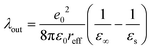(20)
where εs and ε are the static and the optical dielectric constants of water, respectively, and reff is the effective radius of the complex; the other symbols have their usual meaning. In the literature, there is some discussion about a possible effect of the image charge on the energy of solvated ions. According to recent simulations,39 in aqueous solutions, the image interactions are strongly screened by the water molecules; therefore, we have neglected them in this equation.

The effective radius reff was estimated as suggested in ref. 44: according to the Born formula, the free energy of solvation for a spherical ion is: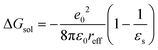(21)
We have calculated the solvation energies of the various reactants from PCM and then chosen reff in such a way that the value obtained from formula (21) agreed with the former. The results are shown in Table 1.

Table 1 Effective radius reff, the bond breaking energy D, the energy of reorganization λout of the outer sphere, and the activation energy ΔEact at zero overpotential. All energies are in eV
[Cu(H2O)5]2+ [Cu(H2O)4Cl2]+
r eff 3.4 2.5
D 1.35 0.2
λ out 1.14 1.56
ΔEact 0.62 0.44

The bond breaking energy D was estimated from the following reactions:

 [Cu(H2O)5]2+ + e− → [Cu(H2O)3]+ + 2H2O (22)
 [Cu(H2O)3Cl2] + e− → [Cu(H2O)2Cl2]− + H2O (23)

The two parameters D and λout characterize the reorganization of the reactants during electron transfer. In particular, they determine the energy of activation, which can be calculated from eqn (19) and (18). The corresponding values have been collected in Table 1. Previously, Blumberger30 made an attempt to address the first step of reduction of [Cu(H2O)5]2+ in terms of asymmetric reorganization (λRedλOx), despite the breaking of bonds between the central ion and water molecules in the first solvation shell. Using the data reported in previous work30 and the formula from ref. 44 to calculate the averaged reorganization energy, a value of 0.46 eV can be obtained for the activation barrier, which is close to our estimate (0.62 eV). Our value is somewhat lower than that of 0.77 eV obtained by Pinto et al.33 from classical molecular dynamics. The same group had obtained an activation energy of 0.68 eV for the second step, so that the first electron transfer would be slow and rate-determining.

Halley et al.45 have measured an activation energy of ΔEact = 0.33 ± 0.05 eV for the first electron transfer step of the pure aqua complex, together with an unusually low pre-exponential factor of about 102 cm s−1. Our theoretical value is substantially lower. The difference may be due to a compensation effect between the activation energy and the pre-exponential factor in the experiments because of the limited temperature range. Alternatively, it could be caused by the neglect of quantum tunneling in our calculations, which would produce the same effect, or to other shortcomings in our model.

The complex with two Cl ions has the lower activation energy. Since its equilibrium potential is similar to that of the pure aqua complex, it offers a favorable reaction path, which would explain the catalytic effect of chloride ions on copper deposition. Unless the deposition step Cu+ + e → Cu, which we have not investigated, is also catalyzed, this would make this deposition step rate-determining. As can be seen from Table 1, the solvent reorganization energy for [CuCl2] is larger compared with that of a pure Cu2+ aqua complex, therefore the catalytic effect results from a smaller intramolecular reorganization for the aquachloro complex.

These findings are in line with the work of Nagy et al.:3 these authors performed experimental work in perchloric acid. In the absence of chloride ions, the first electron transfer in the reduction of copper was found to be rate-determining. Even small amounts of chloride ions accelerated the first electron transfer, but had no effect on the second step, which became rate-determining as the chloride ion concentration was increased. They explained the catalysis of the first step through an increase of the overlap of the reactant with the copper electrode in the presence of chloride ions, while we explain it through the reduction of the energy of reorganization. These two explanations do not exclude each other. The work of Markovic et al.34 further supports our conclusions: they noted that the overpotential deposition of copper on platinum is slow in pure perchloric acid, but substantially enhanced by the presence of chloride ions. They suggested that the chloride ion perturbs the solvation sphere of the copper ions and thereby accelerates the reaction, which qualitatively agrees with our conclusions.

There is a plethora of articles on copper deposition, and an exhaustive citation, let alone discussion, is outside the scope of this article, in which copper deposition is treated as an example. Many studies have been conducted in sulphuric acid, e.g.ref. 2, 35, 37 and 46, which also enhances the deposition rate compared to that in perchloric acid.3 Other studies focused on high chloride ion concentrations, where complexes with three or more chloride ions can be formed,36,46 and where the disproportionation of Cu+ might also play a role. All studies agree that small and intermediate concentrations of chloride ions catalyze copper deposition. Most studies also agree that in the absence of chloride ions, the deposition occurs in two steps, where the first electron transfer determines the rate. However, sometimes, a direct two-electron transfer is proposed.46 This would require an energy of reorganization four times larger than that for a single electron transfer, and is therefore unlikely.38

We tentatively conclude that our results explain the experiments in perchloric acid and at chloride ion concentrations that are not so high that complexes with three or more chloride ions may form. A theoretical explanation of the majority of the experimental data would have to include the elucidation of the effect of sulphate ions on the reaction.

## 7 Conclusions

We have developed a model Hamiltonian for the effect of ion pairing on an outer sphere electron transfer reaction, which was solved in the weak adiabatic limit, i.e. on the Marcus and Hush level.10,11 The formal expression for the activation energy, including the effect of bond breaking, is not affected by ion pairing. However, the presence of the ion modifies the energy of reorganization.

As an application, we have investigated copper deposition from aqueous solutions, focusing on the transfer of the first electron. For this purpose, we have performed DFT calculations for various aqueous copper complexes with and without chloride ions. Our results suggest that the reaction: CuCl2·aq + e → CuCl2·aq has a much lower activation energy than the reaction for the pure aqua complex, and may be responsible for the experimentally observed catalysis of copper deposition by chloride ions.

In summary, we have developed a framework for investigating the effect of ion pairing on electron transfer, and a first application to copper deposition has given promising results.

## Conflicts of interest

There are no conflicts to declare.

## Appendix: technical details of the DFT calculations

The quantum chemical calculations were performed at the DFT level with the hybrid exchange correlation functional B3LYP as implemented in the Gaussian 16 program suite.40 The orbitals of the Cu, Cl, O and H atoms were described by the standard basis set of triple-zeta quality augmented by diffusive and polarization functions, 6-311++g(dp). The initial geometry of the complex species was obtained at the same DFT level but using a smaller basis set. In these calculations, a basis set of double-zeta quality was employed to describe the valence orbitals of the Cu atom, while the effect of inner electrons was included in the effective core potential developed by Hay and Wadt (LanL2).40 The standard basis set 6-31g(d,p) was used to describe the electrons in the Cl, O and H atoms. The open shell systems were treated in terms of the unrestricted formalism. The geometry of complex forms was fully optimized without symmetry restrictions. Water molecules were included directly into the first and second coordination spheres; the rest of the aqueous medium was addressed by the PCM (polarized continuum model).40 A value of 78 was used for the static dielectric constant of the electrolyte solutions. Non-electrostatic (cavitation, dispersion and repulsion) contributions to the solvation free energy were calculated as well. The solvation effects were found to be important in further calculations. Zero-point energy correction and vibrational contributions to the free energy were neglected when estimating the equilibrium potentials. The stability of Kohn–Sham orbitals was checked with the help of a standard routine in the Gaussian 16 package. The ChemCraft42 program was used for the visualization of geometry and orbitals. A similar model approach was employed earlier to investigate the reactivity of In(III)41 and Fe(III)43 aqua- and aquahyrdoxocomplexes.

## Acknowledgements

We gratefully acknowledge the financial support provided by the Deutsche Forschungsgemeinschaft (Schm344/49-1). W. S., E. S. and P. Q. thank CONICET for the continued support. R. N. thanks RFBR (project No 20-0300772). The authors acknowledge the support provided by the state of Baden-WŸrttemberg through bwHPC and the Deutsche Forschungsgemeinschaft through grant no. INST 40/467-1 FUGG (JUSTUS cluster). E. C. and P. Q. acknowledge the financial support of the Agencia Nacional de Promoción Científica y Tecnológica (PICT 2017 – Nro 1342).

## References

1. J. W. Dini, Electrodeposition, The Materials Science of Coatings and Substrates, Noyes Publications, 1993 Search PubMed; Electrodeposition of Copper in Modern Electroplating, ed. M. Schlesinger and M. Paunovic, Wiley, New York, 4th edn, 2000 Search PubMed.
2. L. Bonou, M. Eyraud, R. Denoyel and Y. Massiani, Electrochim. Acta, 2002, 47, 4139 CrossRef CAS.
3. Z. Nagy, J. P. Blaudeau, N. C. Hung, L. A. Curtiss and D. J. Zurawski, J. Electrochem. Soc., 1995, 142, L87 CrossRef CAS.
4. J. M. Saveant, J. Am. Chem. Soc., 1987, 109, 6788 CrossRef CAS.
5. J. M. Saveant, Acc. Chem. Res., 1993, 26, 455 CrossRef CAS.
6. W. Schmickler, J. Electroanal. Chem., 1986, 204, 31 CrossRef CAS.
7. J. Grimminger, S. Bartenschlager and W. Schmickler, Chem. Phys. Lett., 2005, 416, 316 CrossRef CAS.
8. P. W. Anderson, Phys. Rev., 1961, 124, 41 CrossRef CAS.
9. D. M. Newns, Phys. Rev., 1969, 178, 1123 CrossRef CAS.
10. R. A. Marcus, J. Chem. Phys., 1956, 24, 966 CrossRef CAS.
11. N. S. Hush, Trans. Faraday Soc., 1961, 57, 557 RSC.
12. A. M. Kuznetsov, Charge Transfer in Physics, Chemistry and Biology, Gordon & Breach, Reading, 1995 Search PubMed.
13. W. Schmickler, Chem. Phys. Lett., 1995, 237, 152 CrossRef CAS.
14. R. R. Dogonadze and A. M. Kuznetsov, J. Electroanal. Chem., 1975, 65, 545 CrossRef CAS.
15. M. T. M. Koper and G. A. Voth, Chem. Phys. Lett., 1998, 282, 100 CrossRef CAS.
16. W. Schmickler, Chem. Phys. Lett., 2000, 317, 458 CrossRef CAS.
17. A. Ignaczak and W. Schmickler, Chem. Phys., 2002, 282, 147 CrossRef.
18. H. M. Lee, S. K. Min, E. C. Lee, J.-H. Min, S. Odde and K. S. Kimb, J. Chem. Phys., 2005, 122, 064314 CrossRef PubMed.
19. V. S. Bryantsev, M. S. Diallo, A. C. T. van Duin and W. A. Goddard III, J. Phys. Chem., 2008, 112, 9104 CrossRef CAS PubMed.
20. H.-B. Yi, F.-F. Xia, Q. Zhou and D. Zeng, J. Phys. Chem. A, 2011, 115, 4416 CrossRef CAS PubMed.
21. F.-F. Xia, H.-B. Yi and D. Zeng, J. Phys. Chem., 2009, 113, 14029 CrossRef CAS PubMed.
22. F.-F. Xia, H.-B. Yi and D. Zeng, J. Phys. Chem. A, 2010, 114, 8406 CrossRef CAS PubMed.
23. J. Chaboy, A. Munoz-Paez, P. Merkling and E. Sanchez Marcos, J. Chem. Phys., 2006, 124, 64509 CrossRef PubMed.
24. A. Pasquarello, I. Petri, P. Salmon, O. Parisel, R. Car, E. Toth, D. Powell, H. Fischer, L. Helm and A. Merbach, Science, 2001, 291, 856 CrossRef CAS PubMed.
25. P. Frank, M. Benfatto and M. Qayym, J. Chem. Phys., 2018, 148, 204302 CrossRef PubMed.
26. G. La Penna, V. Minigozzi, S. Morante, G. C. Rossi and F. Stellato, J. Chem. Phys., 2015, 143, 124508 CrossRef CAS PubMed.
27. P. Frank, M. Benfatto, M. Qayyam, B. Hedman and K. O. Hodgson, J. Chem. Phys., 2015, 142, 084310 CrossRef PubMed.
28. M. Benfatto, P. D’Angelo, S. D. Longa and N. V. Pavel, Phys. Rev. B: Condens. Matter Mater. Phys., 2002, 65, 174205 CrossRef.
29. J. Blumberger, L. Bernasconi, I. Tavemelli, R. Vuilleumier and M. Sprik, J. Am. Chem. Soc., 2003, 126, 3928 CrossRef PubMed.
30. J. Blumberger, J. Am. Chem. Soc., 2008, 130, 16065 CrossRef CAS PubMed.
31. X. Liu, X. Lu, E. Jan Meijer and R. Wang, Phys. Chem. Chem. Phys., 2010, 36, 10801 RSC.
32. Standard Potentials in Aqueous Solutions, ed. A. Bard, R. Parsons and J. Jordan, Marcel Dekker, New York, 1985 Search PubMed.
33. L. M. C. Pinto, P. Quaino, E. Santos and W. Schmickler, ChemPhysChem, 2014, 15, 132 CrossRef CAS PubMed.
34. N. M. Markovid, H. A. Gasteiger and P. N. Ross, Jr., Langmuir, 1996, 11, 4098 CrossRef.
35. W. Shao, G. Pattanaik and G. Zangari, J. Electrochem. Soc., 2007, 154, D201 CrossRef CAS.
36. H. Zhao, J. Chang, A. Boika and A. J. Bard, Anal. Chem., 2013, 85, 7696 CrossRef CAS PubMed.
37. A. P. Omullane, A. K. Neufeld and A. M. Bond, J. Electrochem. Soc., 2008, 155, D538 CrossRef CAS.
38. W. Schmickler and E. Santos, Interfacial Electrochemistry, Springer Verlag, Berlin, 2nd edn, 2010 Search PubMed.
39. I. L. Geada, H. Ramezani-Dakhel, T. Jamil, M. Sulpizi. and H. Heinz, Nat. Commun., 2018, 9, 716 CrossRef PubMed.
40. M. J. Frisch, G. W. Trucks and H. B. Schlegel, et al., Gaussian 16, Revision B.01, Gaussian, Inc., Wallingford CT, 2016 Search PubMed.
41. R. R. Nazmutdinov, T. T. Zinkicheva, G. Tsirlina and Z. Kuzminova, Electrochim. Acta, 2005, 50, 4888–4896 CrossRef CAS.
42. Chemcraft – graphical software for visualization of quantum chemistry computations, https://www.chemcraftprog.com.
43. R. R. Nazmutdinov, T. T. Zinkicheva, M. E. Kolpakov and A. F. Dresvyannikov, J. Struct. Chem., 2019, 60, 1226 CrossRef CAS.
44. R. R. Nazmutdinov, Russ. J. Electrochem., 2002, 38, 111 CrossRef CAS.
45. J. W. Halley, B. B. Smith, S. Walbran, L. A. Curtiss, R. O. Ridney, A. Sutjianto, N. C. Hung, R. M. Yonko and Z. Nagy, J. Chem. Phys., 1999, 110, 6538 CrossRef CAS.
46. C. T. J. Low, C. Ponce de Leon and F. C. Walsh, Trans. IMF, 2014, 92, 1 CrossRef.
 This journal is © the Owner Societies 2020